Next: 1.2 Propositions Up: 1. Notation, Undefined Concepts Previous: 1. Notation, Undefined Concepts   Index

# 1.1 Sets

The ideas discussed in this chapter (e.g. set, proposition, function) are so basic that I cannot define them in terms of simpler ideas. Logically they are undefined concepts, even though I give definitions for them. My definitions'' use undefined words (e.g. collection, statement, rule) that are essentially equivalent to what I attempt to define. The purpose of these definitions'' and examples is to illustrate how the ideas will be used in the later chapters. I make frequent use of the undefined terms true'', false'', and there is''. It might be appropriate to spend some time discussing various opinions about the meaning of  truth" and  existence" in mathematics, but such a discussion would be more philosophical than mathematical, and would not be very relevant to anything that follows. If such questions interest you, you might enjoy reading Philosophy of Mathematics by Benacerraf and Putnam  or the article Schizophrenia in Contemporary Mathematics by Errett Bishop [10, pp 1-10]

Some of the terms and notation used in the examples in this chapter will be defined more precisely later in the notes. In this chapter I will assume familiar properties of numbers that you have used for many years.

1.1   Definition (Set.) A set is a collection of objects. A small set is often described by listing the objects it contains inside curly brackets, e.g.,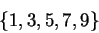denotes the set of positive odd integers smaller than ten.

1.2   Notation (.) A few sets appear so frequently that they have standard names: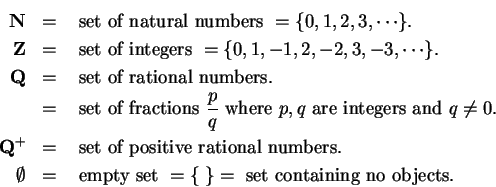1.3   Notation (.) If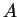is a set and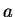is an object, we write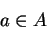(read this asis in") to mean thatis an object in, and we write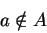(read this asis not in") to mean thatis not in.

1.4   Example. Thus we have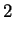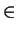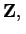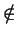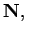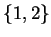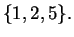(1.5)

To see why (1.5) is true, observe that the only objects inare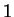,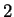, and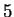. Sinceit follows that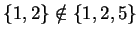.

1.6   Definition (Subset,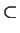.) Letand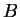be sets. We say thatis a subset ofand writeif and only if every object inis also in.

1.7   Example.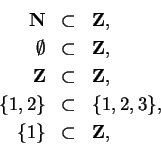are all true statements. However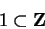(1.8)

is not a statement, but an ungrammatical phrase, sincehas only been defined whenandare sets, andis not a set.

1.9   Definition (Set equality.) Two setsandare considered to be the same if and only if they contain exactly the same objects. In this case we write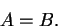Thus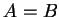if and only ifand.

1.10   Example.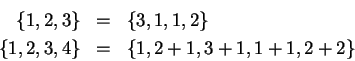Next: 1.2 Propositions Up: 1. Notation, Undefined Concepts Previous: 1. Notation, Undefined Concepts   Index